# MP Board Class 6th Maths Solutions Chapter 9 Data Handling Ex 9.1

## MP Board Class 6th Maths Solutions Chapter 9 Data Handling Ex 9.1

Question 1.
In a Mathematics test, the following marks were obtained by 40 students. Arrange these marks in a table using tally marks.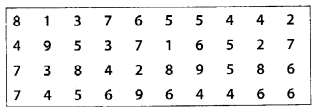(a) Find how many students obtained marks equal to or more than 7.
(b) How many students obtained marks
Solution:(a) 5 + 4 + 3 = 12 students obtained marks equal to or more than 7.
(b) 2 + 3 + 3 = 8 students obtained marks below 4.

Question 2.
Following is the choice of sweets of 30 students of Class VI.
(a) Arrange the names of sweets in a table using tally marks.
(b) Which sweet is preferred by most of the students?
Solution: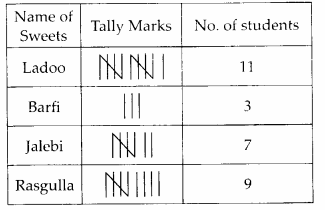(b) Ladoo is preferred by most of the students.Question 3.
Catherine threw a dice 40 times and noted the number appearing each time as shown below: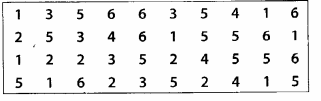Make a table and enter the data using tally marks. Find the number that appeared
(a) The minimum number of times.
(b) The maximum number of times.
(c) Find those numbers that appear an equal number of times
Solution: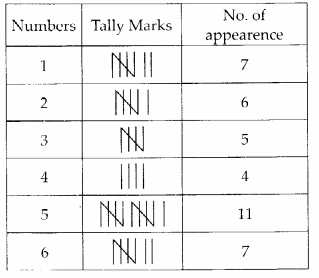(a) 4 appeared minimum number of times.
(b) 5 appeared maximum number of times.
(c) 1 and 6 appeared equal number of times.Question 4.
Following pictograph shows the number of tractors in five villages.Observe the pictograph and answer the following questions.
(i) Which village has the minimum number of tractors?
(ii) Which village has the maximum number of tractors?
(iii) Flow many more tractors village C has as compared to village B.
(iv) What is the total number of tractors in all the five villages?
Solution:
(i) Village D has the minimum number of tractors.
(ii) Village C has the maximum number of tractors.
(iii) Village C has 8 – 5 = 3 more tractors than village B.
(iv) Total number of tractors = 6 + 5 + 8 + 3 + 6 = 28Question 5.
The number of girl students in each class of a co-educational middle school is depicted by the pictograph: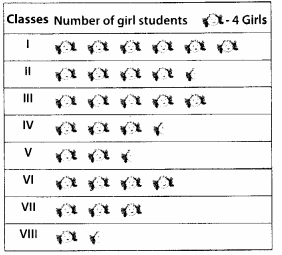Observe this pictograph and answer the following questions:
(a) Which class has the minimum number of girl students?
(b) Is the number of girls in Class VI less than the number of girls in Class V?
(c) Flow many girls are there in Class VII?
Solution:(a) Class VIII has the minimum number of girl students.
(b) No, the number of girls in Class VI is greater than the number of girls in Class V.
(c) There are 12 girls in Class VII.Question 6.
The sale of electric bulbs on different days of a week is shown below: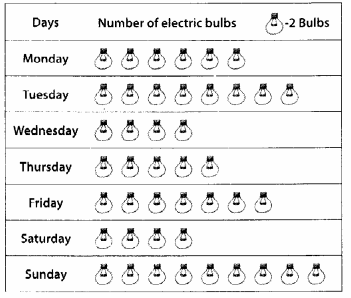Observe the pictograph and answer the following questions:
(a) How many bulbs were sold on Friday?
(b) On which day were the maximum number of bulbs sold?
(c) On which of the days same number of bulbs were sold?
(d) On which of the days minimum number of bulbs were sold?
(e) If one big carton can hold 9 bulbs. How many cartons were needed in the given week ?
Solution:(a) Number of bulbs sold on Friday is 14.
(b) Maximum number of bulbs were sold on Sunday.
(c) Same number of bulbs were sold on Wednesday and Saturday.
(d) Minimum number of bulbs were sold on Wednesday and Saturday.
(e) The total number of bulbs were sold in the given week = 86
Number of cartons required for 9 bulbs = 1
∴ Number of cartons required for 86 bulbs = 86 ÷ 9 = 9.55 = 10
Therefore, 10 cartons were needed in the given week.Question 7.
In a village six fruit merchants sold the following number of fruit baskets in a particular season: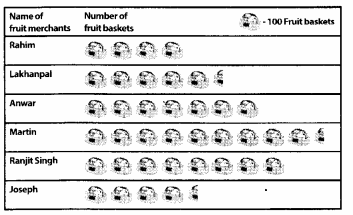Observe this pictograph and answer the following questions:
(a) Which merchant sold the maximum number of baskets?
(b) How many fruit baskets were sold by Anwar?
(c) The merchants who have sold 600 or more number of baskets are planning to buy a godown for the next season. Can you name them?
Solution: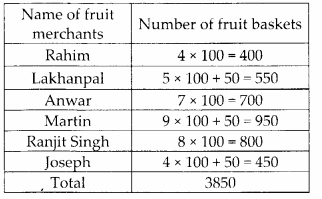(a) Martin sold the maximum number of baskets.
(b) 700 fruit baskets were sold by Anwar.
(c) Anwar, Martin and Ranjit Singh have sold more than 600 baskets.